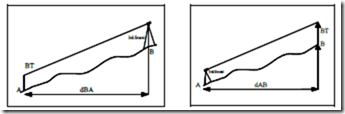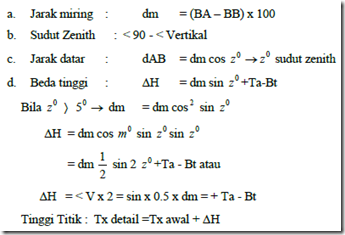Home > English > Measurement Situation How Polar Coordinates

Measurement Situation How Polar Coordinates

Types of tasks: Situation Measurement Method of Polar Coordinates Method Without Tacheometry Magnetism.
Measurement tools:

• Theodolites plane TL 6 DE
• The stand
• Rol meter
• Hammer
• Signs measure
• List of measurement
• Data board
• Peg / nail

Work safety :
1. Check the measurement tools before being taken to the place of measurement.
2. Check the location of the measurement of the state / vehicle traffic so as not to interfere with the measurement.
3. Doing good cooperation with friends so that the measurement accuracy.
4. Cross the road waiting for a vehicle / traffic safety.
5. Protect theodelite plane of the sun.
6. Clean the equipment before it is returned.

Work steps :
1. Note the location of the measurement and sketching measurement situations.
2. Determine the best place to stand so that all points are measured can terbidik all (not blocked).
3. Define the measurement points by 25 points with a marked spike in the streets or boundaries of measurement.
4. Set the air above a predetermined point.
5. Aim binoculars to point P, by setting the degree of flattening at 00 0 ‘0 “.
6. Read the vertical angle of the regular reading and recording on the list (when reading the first vertical angle cross thread middle dihimpitkan on reading the signs above the high point P1 plane).
8. Turn the binoculars and membidikan back to point P1 and then read the reading remarkable flat angle and vertical angle remarkable.
9. After the restore the position of binoculars with mengimpitkan cross thread in the middle of the same signs with high aircraft, (Ta = BT) or higher may not equal plane with the central thread (Ta ≠ BT) and then read the vertical angle (plain text), and then read upper and lower thread yarn (BA and BB).
10. Move the binoculars with mengimpitkan cross thread in the middle of the same signs with numbers higher plane, then read the vertical angle (plain text), and then read the thread top and bottom threads.
11. Feedback binoculars and rotated clockwise and membidikan back to point P1 to read the flat and vertical angles outstanding.
12. In the same way do the work steps as above for the following points, until the end of measurement.

Calculations by means tachymetri:"Tasikmalaya, Indonesia"